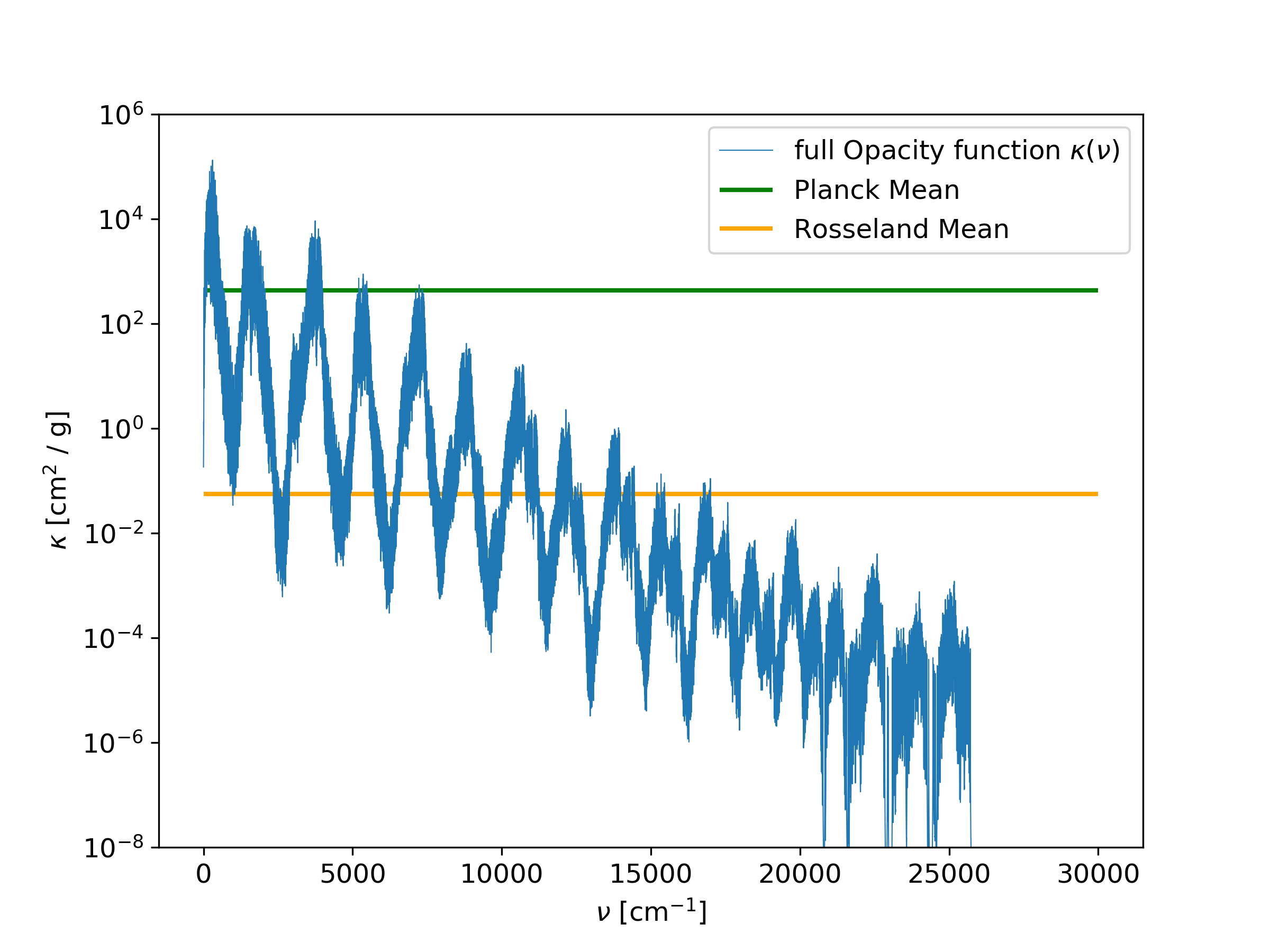# Planck and Rosseland Means¶

With the doMean option in the param.dat file, the Plank and Rosseland means, $$\kappa_P$$ and $$\kappa_R$$ respectively:

(8)$\kappa_P = \frac{\int_0^\infty \kappa B_{\nu}(T) d\nu}{\int_0^\infty B_{\nu}(T) d\nu},$

and

(9)$\kappa_R = \left( \frac{\int_0^\infty \kappa^{-1} \frac{\partial B_{\nu}(T)}{\partial T} d\nu} {\int_0^\infty \frac{\partial B_{\nu}(T)}{\partial T} d\nu} \right)^{-1}$

can be calculated, where the infinity integral is truncated to the specified wavenumber limits, and $$d\nu$$ is equal to dnu set in the param.dat file.

Note that the denominators in $$\kappa_P$$ and $$\kappa_R$$ can be computed analytically as

(10)$\int_0^\infty B_{\nu}(T) d\nu = \frac{\sigma T^4} {\pi}$

and

(11)$\int_0^\infty \frac{\partial(B_{\nu}(T))}{ \partial (T)} d\nu = \frac{4 \sigma T^3} {\pi}.$

Therefor, it is usefull to compare those analytic results to the numerical integration

(12)$\int_0^\infty B_{\nu}(T) d\nu = \sum_i B_{\nu}(T) dnu$

and

(13)$\int_0^\infty \frac{\partial(B_{\nu}(T))}{ \partial (T)} d\nu = \sum_i \frac{\partial(B_{\nu}(T))}{ \partial (T)} dnu$

The results of the Planck and Resseland means are stored in the file Out<name>_mean.dat, together with the analytic and numerical expressions (10) to (13). If the numerical expressions deviate strongly from the analytical expression, then it is a hint that the wavenumber resolution is not set fine enough.

An example of the Planck and Rosseland means is shown in Fig. 11.

Relevant parameters for this example:
• doStoreFullK = 1

• doMean = 1Fig. 11 Planck and Rosseland means